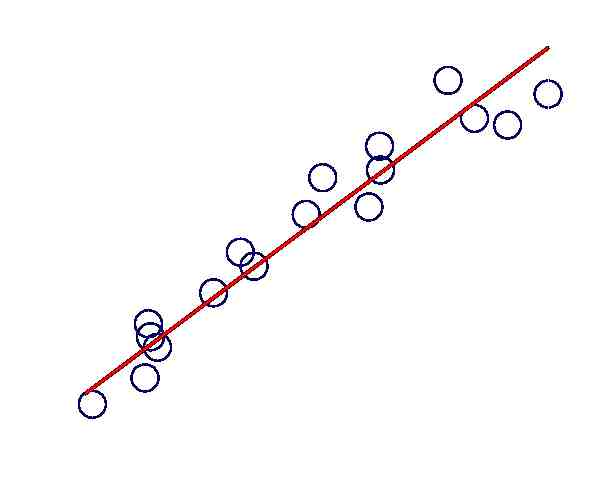# Linear Regression Calculator

Instructions: Perform a regression analysis by using the Linear Regression Calculator , where the regression equation will be found and a detailed report of the calculations will be provided, along with a scatter plot. All you have to do is type your X and Y data. Optionally, you can add a title and add the name of the variables.X data (comma or space separated)Y data (comma or space separated)Type the title (optional)Name of X variable (optional)Name of Y variable (optional)

A linear regression model corresponds to a linear regression model that minimizes the sum of squared errors for a set of pairs $$(X_i, Y_i)$$.

The linear regression equation, also known as least squares equation has the following form: $$\hat Y = a + b X$$, where the regression coefficients $$a$$ and $$b$$ are computed by this regression calculator as follows:

$b = \frac{SS_{XY}}{SS_{XX}}$ $a = \bar Y - \bar X \cdot b$

The coefficient $$b$$ is known as the slope coefficient, and the coefficient $$a$$ is known as the y-intercept.

If instead of a linear model, you would like to use a non-linear model, then you should consider instead a polynomial regression calculator , which allows you to use powers of the independent variable.### Linear regression calculator Steps

The steps to conduct a regression analysis are:

(1) Get the data for the dependent and independent variable in column format.

(2) Type in the data, either in comma separated or space separated format.

(3) Press "Calculate".

### Regression Residuals

How do we assess if a linear regression model is good? You may think "easy, just look at the scatterplot ". In reality, math and statistics tend to go beyond where the eye meets the graph. It is usually risky to rely solely on the scatterplot to assess the quality of the model.

In terms of goodness of fit, one way of assessing the quality of fit of a linear regression model is by computing the coefficient of determination , indicates the proportion of variation that in the dependent variable that is explained by the independent variable.

In linear regression, the fulfillment of the assumptions is crucial so that the estimates of the regression coefficient have good properties (being unbiased, minimum variance, among others).

In order to asses the linear regression assumptions, you will need to take a look at the residuals. For that purpose, you can take a look at our residual calculator .

### Other calculators related to linear regression

You may also be interested in computing the correlation coefficient , or to construct a scatter plot with the data provided.